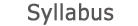2015 - 2016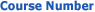0509-1847-03
Calculus 2b
FACULTY OF ENGINEERING | DEPARTMENTS OF ENGINEERING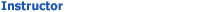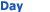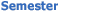Eitan Rosen Wolfson - Engineering 120 Mon 1300-1500 Sem  2
University credit hours:  2.0

Course description
Background: differential and integral calculus 1b, linear algebra.
1. Limit and continuity of functions of two variables, iterated limits, partial derivatives, the chain rule, changing the order of differentiation, implicit functions and their derivatives. Taylor formula, extremum, Lagrange multiplier method.
2. Double and triple integrals, variables changing in double and triple integrals, Jacobian. Polar, cylindrical, and spherical coordinates. Application in calculation of lengths, areas, volumes, moments and centers of mass.
3. Line integrals of the first and second kinds. Green's theorem. Surface integrals of the first and second kinds.
4. Theory of vector fields, operators: gradient, divergence and rotor. Theorems of Gauss and Stokes.

accessibility declaration